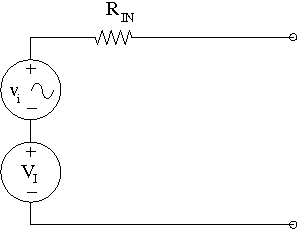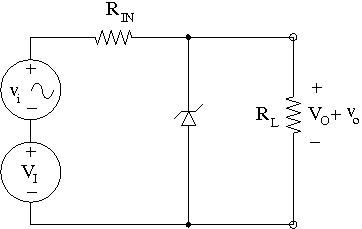## Zener regulator

 A non-ideal voltage source can be modeled by a series combination of an ideal DC voltage source, a resistance, and a small-signal voltage source. The small-signal source is included to represent the noise inherent in the source. This model is illustrated in the figure below:In practice, connecting the non-ideal voltage source to a load may result in undesirable effects due to the noise voltage vo appearing across the load.This problem studies such effects and how a Zener diode may be used to ameliorate the problem. Assume that VI=9.5 V, RIN=1 kΩ , and vi=50.0 mV. In the figure above, calculate the DC output voltage VO , and the output noise voltage vo for two values of the load resistance. 1.For RL=2 kΩ , the value of VO (in Volts) is: 2.and the value of vo (in Volts, to at least 3 decimal places) is: 3.For RL=4 kΩ the value of VO (in Volts) is: 4.and the value of vo (in Volts, to at least 3 decimal places) is: Now, we can insert a Zener diode into the circuit, as shown below. The Zener diode is a nonlinear device and a piecewise-linear approximation to its i-v characteristic is shown graphically below.Again, we calculate vo (i.e. output noise) and VO (i.e. DC output voltage) for RL =2k Ω and RL =4k Ω for this new circuit. Hint: Your first job here is to determine which of the three regions of the piecewise-linear characteristic of the Zener is the one containing the operating point: we suggest you sketch a load line, but be very careful about the signs. Once you have determined where the operating point is, you can model the Zener in the circuit with a series combination of an independent voltage source and a resistor. 5.For RL=2 kΩ the value of VO (in Volts) is: 6.And the value of vo (in Volts, to at least 5 decimal places) is: 7.For RL=4 kΩ the value of VO (in Volts) is: 8.And the value of vo (in Volts, to at least 5 decimal places) is: 9.What is the minimum value of RL , in Ohms, that guarantees that the circuit will operate this way? by servomotor July 28, 2020 We don't have the I-V curve approx. for the Zener diode. It probably give its Vz (at 1 mA in reverse) and the resistance value for that almost perpendicular segment which may be essential to solve the problem. Furthermore, that looks like a homework problem. Can't you sketch the circuit with the circuitlab simulator? You have a problem doing exactly that? by vanderghast July 28, 2020 I agree completely with @vanderghast's comment :) What have you tried already? Where exactly are you stuck? by mrobbins July 28, 2020

CircuitLab's Q&A site is a FREE questions and answers forum for electronics and electrical engineering students, hobbyists, and professionals.

We encourage you to use our built-in schematic & simulation software to add more detail to your questions and answers.

Acceptable Questions:

• Concept or theory questions
• Practical engineering questions
• “Homework” questions
• Software/hardware intersection
• Best practices
• Design choices & component selection
• Troubleshooting

Unacceptable Questions:

• Non-English language content
• Non-question discussion
• Non-electronics questions
• Vendor-specific topics
• Pure software questions
• CircuitLab software support

Please respect that there are both seasoned experts and total newbies here: please be nice, be constructive, and be specific!